# Angle Between Two Lines

Let y = m1x + c1 and y = m2x + c2 be the equations of two lines in a plane where,

```m1 = slope of line 1
c1 = y-intercept made by line 1

m2 = slope of line 2
c2 = y-intercept made by line 2```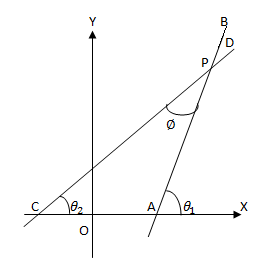```<BAX = θ1
<DCX = θ2

∴ m1 = tan θ1 and
m2 = tan θ2                                        ```
Let the angle between the lines AB and CD be Ø (<APC) then,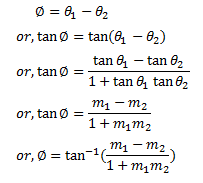Also if we consider <APD as the angle between lines,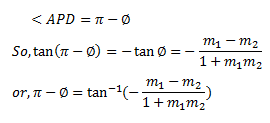Hence, the angle between two lines is,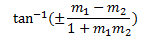Condition for perpendicularity

The two lines are perpendicular means. Ø = 90°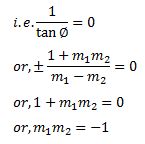Thus, the lines are perpendicular if the product of their slope is -1.

Condition for parallelism

The two lines are perpendicular means, Ø = 0°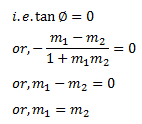Thus, the lines are parallel if their slopes are equal.

### Angle Between Two Lines Examples

1. Find the angle between the lines 2x-3y+7 = 0 and 7x+4y-9 = 0.

Solution:

Comparing the equation with equation of straight line, y = mx + c,

Slope of line 2x-3y+7=0 is (m1) = 2/3

Slope of line 7x+4y-9=0 is (m2) = -7/4

Let, Ø be the angle between two lines, then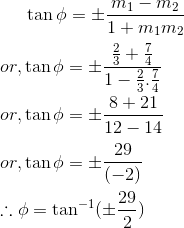2. Find the equation of line through point (3,2) and making angle 45° with the line x-2y = 3.

Solution:

Let m be the slope of the required line passing through (3,2). So, using slope point form, its equation is

y-2 = m(x-3) ——— (i)

Slope of line x-2y = 3 is 1/2.

Since, these lines make an angle of 45° so,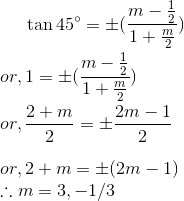Substituting values of m in equation (i), we get

y-2 = 3(x-3) and y-2 = -(x-3)/3

or, 3x-y-7 = 0 and x+3y-9 = 0 are the required equations of line.